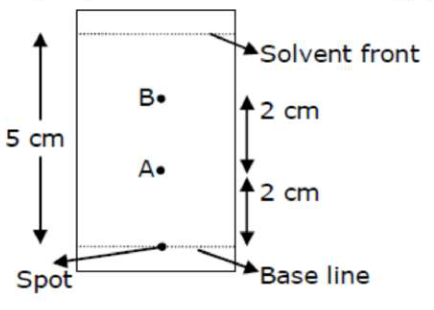# Using the provided information in the following paper chromatogram:Question:

Using the provided information in the following paper chromatogram:Fig: Paper chromatography for compounds A and B

The calculated $R_{f}$ value of $A$ ________________ $\times 10^{-1}$

Solution:

(4)

$\mathrm{R}_{\mathrm{f}}=\frac{\text { Dis tan ce travelledby compound }}{\text { Dis tan ce travelled by solvent }}$

On chromatogram distance travelled by compound is $\rightarrow 2 \mathrm{~cm}$

Distance travelled by solvent $=5 \mathrm{~cm}$

So $\mathrm{R}_{\mathrm{f}}=\frac{2}{5}=4 \times 10^{-1}=0.4$